Basic VR Tube Regulator Circuit resistance (RL) if the tube is operated in the normal glow discharge region. ">Custom SearchBasic VR Tube Regulator Circuit Although we covered the electrical characteristics of the VR tube in chapter 2 of this module, we now need to cover the capabilities and limitations of the VR tube itself. Figure 3-43 shows a basic VR tube regulating circuit. The voltage produced by the source is 150 volts. The VR 90 will provide a constant 90 volts across the load resistance (RL) if the tube is operated in the normal glow discharge region. This means that 60 volts is dropped across RS, which is the series limiting resistance used to limit the current through the VR tube. Figure 3-43. - Basic VR tube regulator.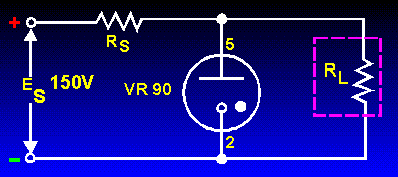Since the operating limits of a VR tube are determined by its maximum and minimum currents, circuits using such tubes should be designed to allow maximum variations in current above and below the normal point of operation. The normal point of operation, which allows maximum variation in current, must be midway between the current limits of the tube. This median current is called Imean. and can be calculated by the use of the following equation: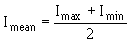We can determine the mean current for the VR90-40 as shown in figure 3-44 by using the following values: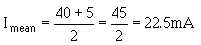To calculate the value of series dropping resistance RS, we use the following equation: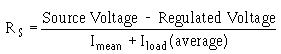If the average current flowing through the load of figure 3-44 is 100 milliamperes, we can find the series dropping resistance in the following manner: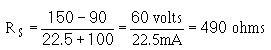Figure 3-44. - Simplified VR tube regulator.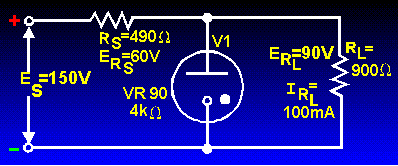According to Ohm's law, the value of the load resistance for this circuit figure will be 900 ohms if a current of 100 milliamperes flows through RL. The internal resistance of the VR tube can be calculated in a similar manner. With 22.5 milliamperes flowing and 90 volts dropped across the VR tube, its resistance is 4 kilohms. To determine the voltage regulation in the circuit for figure 3-44, assume a constant supply voltage of 150 volts and a variable load resistance. If the value of RL were to decrease to 857 ohms, the load current would increase to approximately 105 milliamperes to maintain 90 volts across the load resistance. RS must drop 60 volts. To do so requires a current of 122.5 milliamperes flowing through the series resistance. Since 105 milliamperes is now flowing through the load, the current through the VR tube must decrease from 22.5 milliamperes to 17.5 milliamperes. We will discuss the sequence of events in more detail to help you better understand how the tube current is made to vary. The original load resistance was 900 ohms. Changes in this resistance will not occur instantaneously, but will require some time to vary from 900 ohms to a new value. As resistance of the load begins to decrease, load current begins to increase. The minute increase in load current will flow through the series resistance RS causing a slight increase in ERS. This slight increase in voltage across RS will result in the VR tube voltage dropping slightly. This slight drop in tube voltage will cause a decrease in the ionization of the tube gas, which in turn increases the resistance of the tube. As a result, less current flows through the tube. Note that tube current can decrease only to a value of 5 milliamperes before deionization occurs. Therefore, the load current cannot exceed 117.5 milliamperes, for beyond this value, tube current becomes less than 5 milliamperes and regulation ceases. If load resistance were to increase, load current would decrease. This would result in the VR tube current increasing to maintain a current of 122.5 milliamperes. The VR tube current can only increase to 40 milliamperes. Beyond this value of current, the tube enters the abnormal glow region and tube voltage increases. The upper limit of the VR tube current will occur when load current decreases to a value of 82.5 milliamperes. When load current drops below this value, the VR tube ceases to regulate the load voltage. Therefore, with a constant source voltage but variable load resistance, the limits of regulation will be reached when current in the load exceeds 117.5 milliamperes or drops below 82.5 milliamperes. The VR tube regulator can also compensate for changes in power supply voltage. Under these conditions, the load resistance will remain constant while the power supply voltage will be variable. Refer to figure 3-44 for the following discussion. Assume that the source voltage begins to increase from an original value of 150 volts toward 155 volts. As this voltage increases, current through RS increases from its original value of 122.5 milliamperes. Initially, this additional current is drawn from the load, causing a slight increase in load voltage. This increase in load voltage is felt across the VR tube and causes an increase in tube ionization. This decreases the internal resistance of the VR tube with a resultant increase in tube current. When source voltage reaches 155 volts, current through RS is approximately 133 milliamperes (RS = 490 ohms). Most of the additional current through RS flows through the VR tube. As a result, approximately 33 milliamperes flows through the VR tube, maintaining the load voltage at 90 volts. Since VR tube current decreases as source voltage decreases, tube current will drop below its lower limit of 5 milliamperes at some point. When source voltage drops below 141.4 volts, tube current will be less than 5 milliamperes and regulation will cease. The upper and lower limits of the supply voltage variations that can be allowed and still provide regulation in the circuit are 158.6 volts and 141.4 volts, respectively. Remember that tube voltage varies slightly through its operating range, but this voltage change is less than that which would exist without the use of a VR tube. As the source voltage increases, the current through the VR tube increases. Since the upper limit of tube current is 40 milliamperes, there is a limit in the ability of the tube to regulate increasing voltage. When the supply voltage exceeds 158.6 volts, tube current will be greater than 40 milliamperes and regulation will cease. If the source voltage decreases from 150 volts to 145 volts, only 55 volts must be dropped across the 490-ohm series resistance (RS ) to maintain the load voltage at 90 volts. Current through RS for a 55 volt drop is 112 milliamperes. Since load current is 100 milliamperes, the remaining 12 milliamperes must flow through the VR tube. This represents a decrease in the ionization level of the VR tube, with a resultant increase in tube resistance. Under these conditions, 90 volts will be maintained across the load resistance. VR Tubes Connected in Series In applications where a regulated voltage in excess of the maximum rating of one tube is required, two or more tubes may be placed in series as shown in figure 3-45. Figure 3-45. - VR tubes as voltage dividers.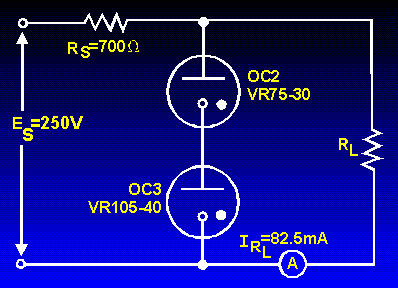In the figure, a VR75-30 and a VR105-40 are shown connected in series. The source voltage is 250 volts, and 82.5 milliamperes flows through the load resistance. Since current through the two VR tubes is common, the limits of regulation are determined by the tube having the smaller current limitations. (In this case, the VR 75-30). In computing Imean for this circuit, Imax and Imin will be 30 milliamperes and 5 milliamperes, respectively. Therefore, the mean current will be 17.5 milliamperes. The value of RS in the figure can be computed using the source voltage of 250 volts and the total current through RS (load current + Imean). Using these values, RS = 700 ohms. Note that the regulated voltage to the load is 180 volts. This provides a regulated voltage greater than would be possible using either VR tube by itself. Another advantage of using VR tubes in series is illustrated in figure 3-46. In this circuit, several values of regulated voltages are obtained from a single power supply. Figure 3-46. - VR tubes as voltage dividers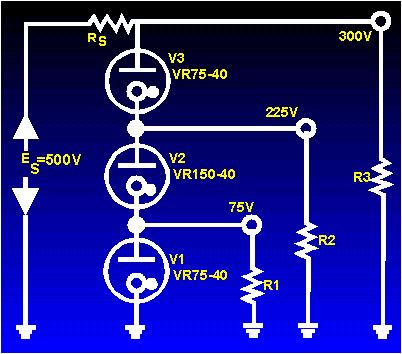The current flowing through V2 in the figure is a combination of the current through R1 and the current through V1. The current through V3, on the other hand, is the sum of the currents through V2 and R2. Since V3 has more current flowing through it than any of the other VR tubes, it places or determines the limit on the maximum current in the VR tube circuit. Since the maximum rating of V3 is 40 milliamperes, the currents through R1 and R2 must be limited to only a few milliamperes, or the rating of V3 will be exceeded and regulation will cease. The obvious advantage in using VR tubes in series is to provide several regulated voltages from a single power supply. The primary disadvantage is in the current limitations. Since it is impossible to have all VR tubes operating about their mean current values, this limits the ability of the circuit to regulate over wide ranges of variations in load resistance or source voltage.Integrated Publishing, Inc. - A (SDVOSB) Service Disabled Veteran Owned Small Business